# AAAI 2023｜香港大学提出Glance-and-Focus网络，有效提升弱监督视频异常检测性能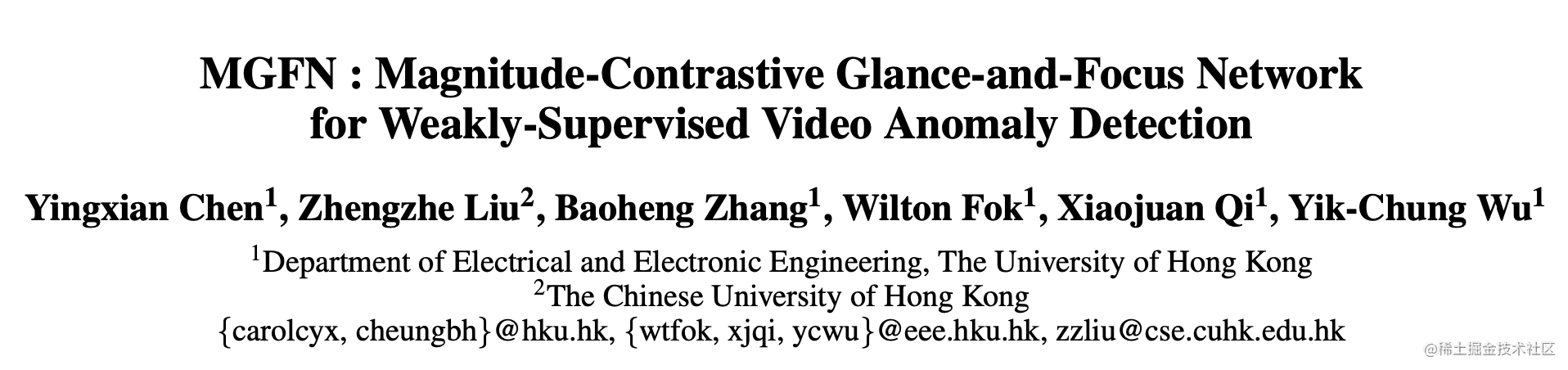## 1.引言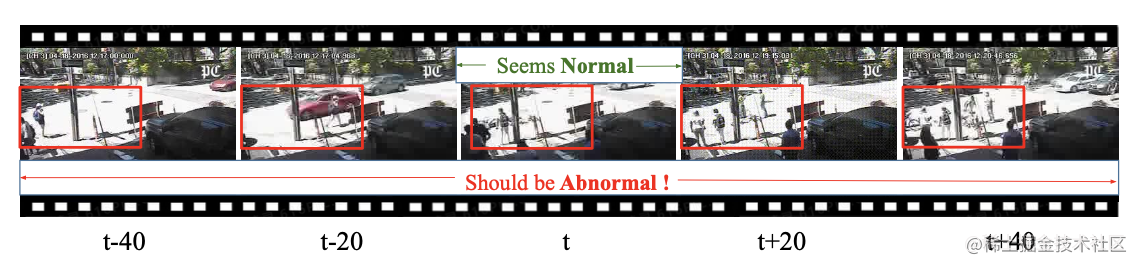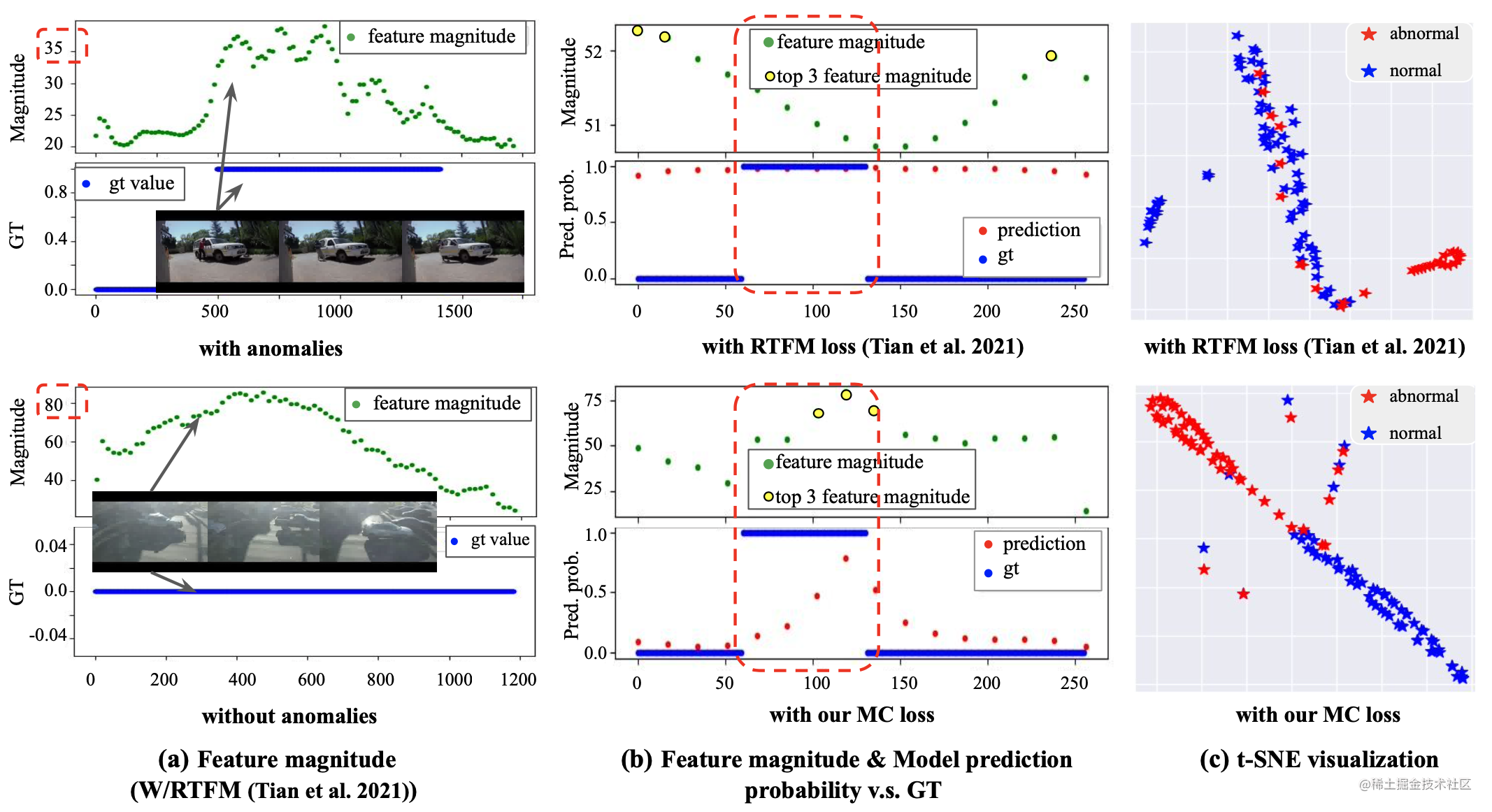## 2.本文方法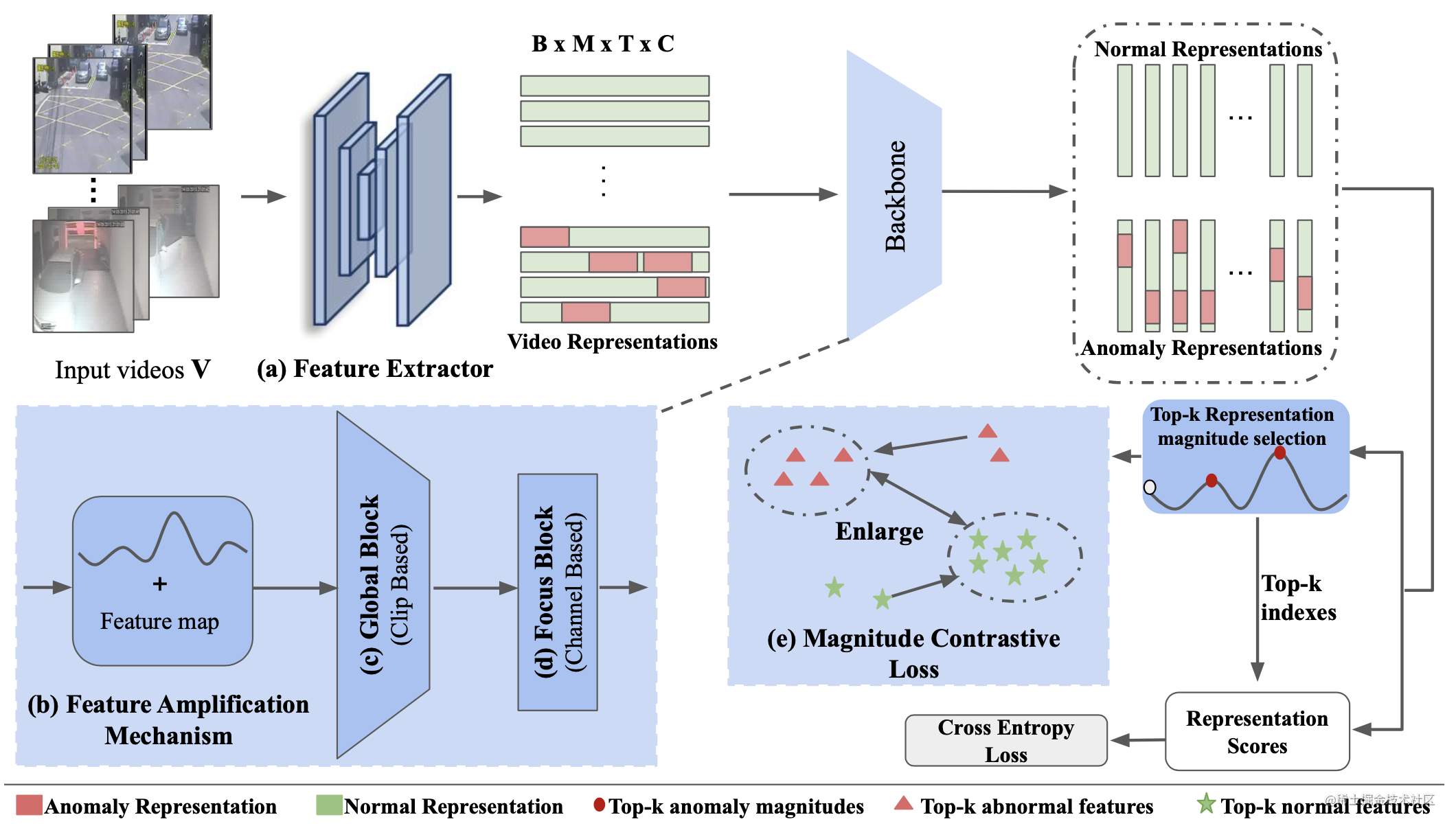### 2.1 特征放大机制（FAM）

FAM模块的构成细节如上图（b）所示，首先显式计算出 $f^{i,t}$ 的特征范数 $M^{i, t}$ 如下所示：

$M^{i, t}=\left(\sum_{c=1}^{C}\left|f^{i, t, c}\right|^{2}\right)^{\frac{1}{2}} \in \mathbb{R}^{1 \times 1 \times P \times 1}$

$f_{F A M}^{i, t}=f^{i, t}+\alpha \operatorname{Conv1D}\left(M^{i, t}\right) \in \mathbb{R}^{1 \times 1 \times P \times C}$

### 2.2 Glance Block(GB)

Glance Block 的架构如下图（a）所示。为了减少计算负担，作者首先使用卷积将特征图维度降低。并通过short-cut卷积将输出特征图转换为 $F_{S c c_{-} G B} \in \mathbb{R}^{B \times T \times P \times C / 32}$，随后构建了一个视频级Transformer来学习视频片段之间的全局相关性。具体来说，作者计算了一个注意力图 $A \in \mathbb{R}^{1 \times T \times T \times P}$ 来明确关联不同的时间片段。

$A^{i, t_{1}, t_{2}}=\sum_{c=1}^{C} Q\left(F_{s c c_{-} G B}^{i, t_{1}, c}\right) K\left(F_{s c c_{-} G B}^{i, t_{2}, c}\right)$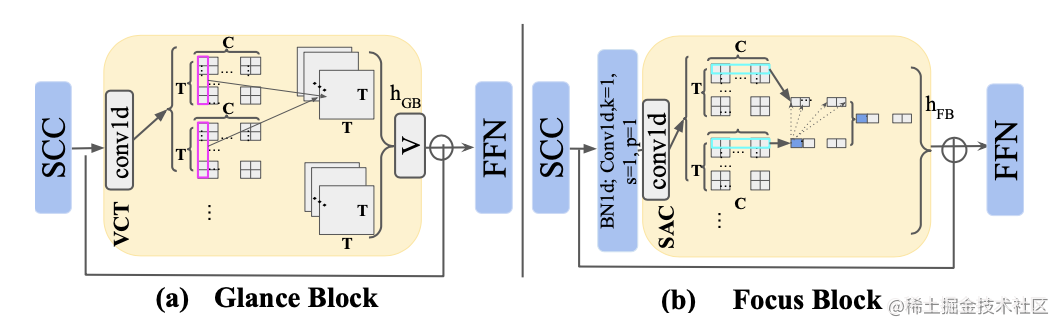Glance Block 包含一个额外的前馈网络 (FFN)，两个全连接层和一个 GeLU 非线性函数以进一步提高模型的表示能力，输出特征图$F_{G B}$j接着被送到Focus Block中提取局部特征。

### 2.3 Focus Block(FB)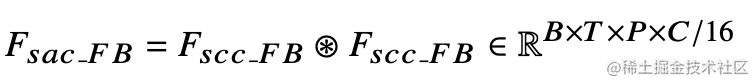$F_{s a c+F B}^{i, t, k_{1}}=\sum_{k_{1}, k_{2}=0}^{C / 16} F_{s c c_{-} F B^{i, t} F_{s c c_{-} F B}^{i, t, k_{2}}}^{i, \mathbb{R}^{1}} \mathbb{1}^{1 \times 1 \times P \times 1}$

### 2.4 Magnitude Contrastive Loss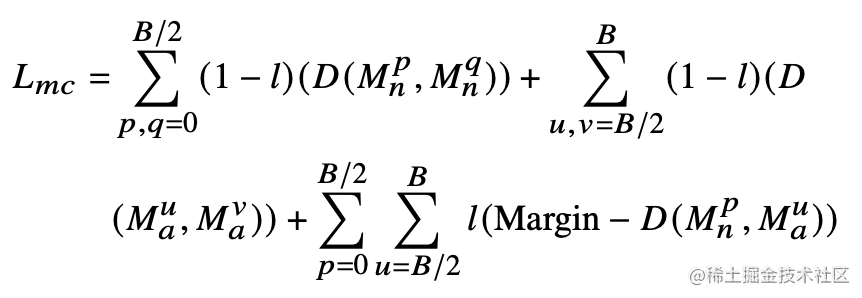## 3.实验效果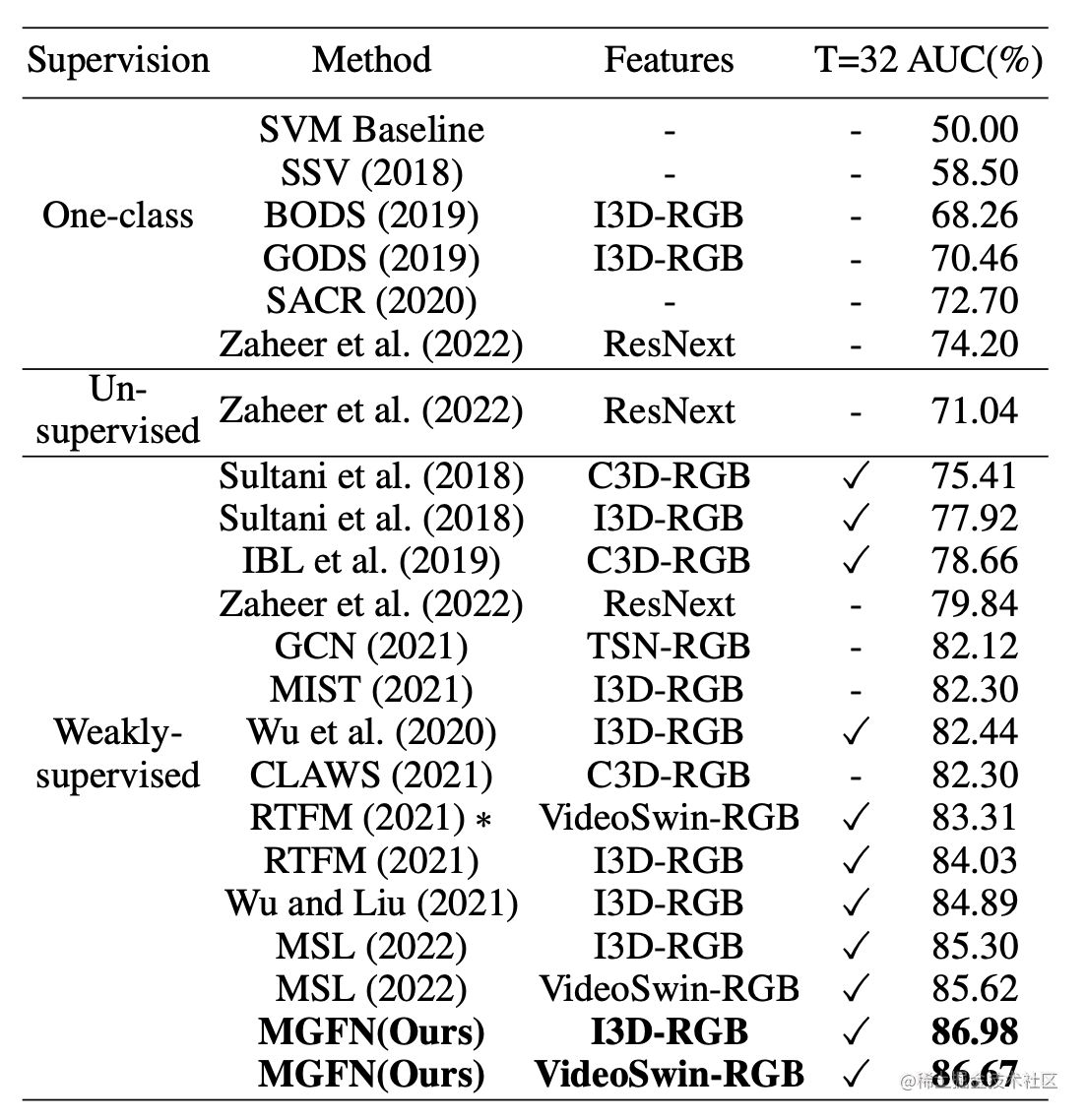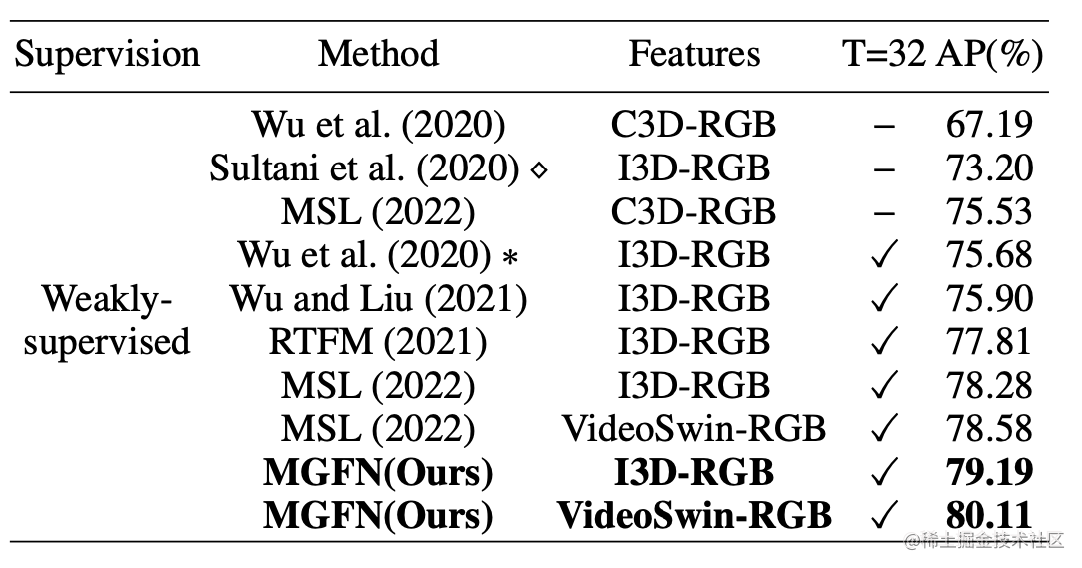## 参考

 Tian, Y.; Pang, G.; Chen, Y.; Singh, R.; Verjans, J. W.; and Carneiro, G. 2021. Weakly-Supervised Video Anomaly Detection With Robust Temporal Feature Magnitude Learning. In Proceedings of the IEEE/CVF International Conference on Computer Vision (ICCV), 4975–4986.